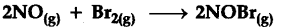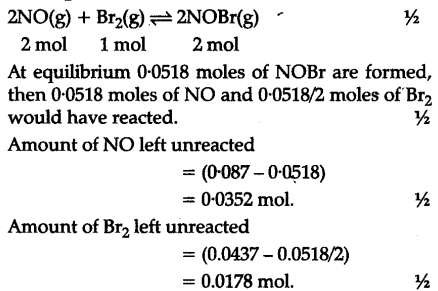# (i) Give two important characteristics of chemical equilibrium

(i) Give two important characteristics of chemical equilibrium.
(ii)Nitric oxide reacts with {{Br}_{2}} and gives nitrosyl bromide as per reaction given below :When 0.087 mol of NO and 0.0437 mol {{Br}_{2}} are mixed in a closed container at constant temperature, 0.0518 mol of NOBr is obtained at equilibrium. Calculate equilibrium amount of NO and\${{Br}_{2}}\$…

(i) Two important characteristics of chemical equilibrium are :
(a) Chemical equilibrium are dynamic in nature.
(b) Chemical equilibrium can be achieved from either of the directions i.e., starting the reaction either by taking the reactants or products.
(ii)